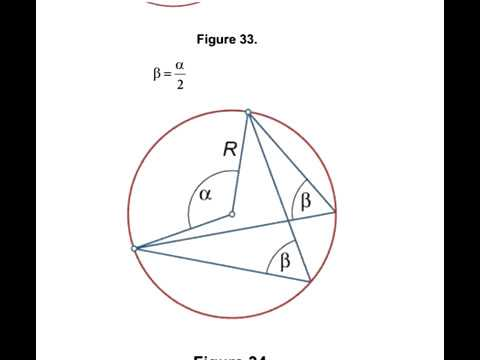All Geometry FormulaEveryone
101An app of formulas for geometry, related to area and perimeter of triangles, rectangles, cercles, sectors, and volume of sphere, cone, cylinder are presented. All Geometry Formula for math students. You will able to search Formulas in this app.

Geometry is a branch of mathematics that deals with shape, size, relative position of figures, and the properties of space. Formulas are used to calculate the length, perimeter, area and volume of various geometric shapes and figures.

This app contain these formulas:

# Trigonometry
# Radian and Degree Measures of Angles
# Definitions and Graphs of Trigonometric Functions
# Signs of Trigonometric Functions
# Trigonometric Functions of Common Angles
# Most Important Formulas
# Reduction Formulas
# Periodicity of Trigonometric Functions
# Relations between Trigonometric Functions
# Adding and Subtraction Formulas
# Double Angle Formulas
# Multiple Angle Formulas
# Half Angle Formulas
# Half Angle Tangent identities
# Transforming of Trigonometric Expressions to Product
# Transforming of Trigonometric Expressions to Sum
# Powers of Trigonometric Functions
# Graphs of Inverse Trigonometric Functions
# Inverse Tangent Function
# Inverse Cotangent Function
# Inverse Secant Function
# Inverse Cosecant Function
# Principal Values of Inverse Trigonometric Functions
# Relations between Inverse Trigonometric Functions
# Trigonometric Equations
Collapse

Review Policy
4.4
101 total
5
4
3
2
1

Updated
October 10, 2019
Size
13M
Installs
10,000+
Current Version
1.0.4
Requires Android
4.0.3 and up
Content Rating
Everyone
Permissions
Offered By
Binary Tuts